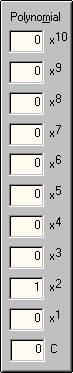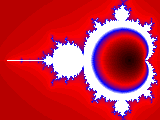PHOTOSWIZZLE Fractals Help - Polynomial## Polynomial` `

The Polynomial controls select which terms of the polynomial are used in the fractal generator equation. Term powers are from 1 through 10 and C (constant) as shown. The value in the box to the left of each term is a multiplier and may be from -999 to +999 inclusive. If the value in the box is 0 or the box is blank the term is not used in the equation.

For example, in the default settings only power 2 term has a non-zero value (1). So the polynomial is x2. A more complex example might be Sample 3, Demon. There the power 5 term has multiplier 5, power 4 has multipler -9, power 2 has multipler 4 and C has multipler 1. So the polynomial is 5x5 -9x4 + 4x2 + 1.

Note that whether the polynomial is used at all in the fractal generation depends on the pattern selected. See Pattern for more details. Here are a few simple variations on the default settings with minor color and position changes:x2x10x10 - 2x7

```
```